Symmetrizing, alternating, wedging

A square matrix decomposes into symmetric and skew-symmetric part. ΤS and ΤA perform these linear projections.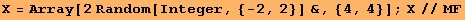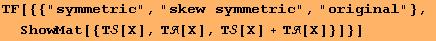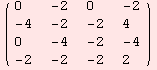symmetric skew symmetric original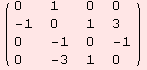The functions ΤS and ΤA that symmetrize and alternate work for tensors of arbitrary rank but with fixed dimension. For instance, consider the (0,4)-tensor X below. Due to its length, we do not show X, but we indicate that S(A(X))=A(S(X))=0.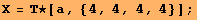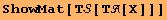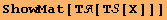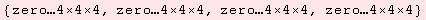DGkernel encodes wedging of two tensors asWhen wedging multiple tensors, use brackets. Have a look at the following simple example.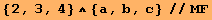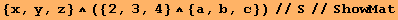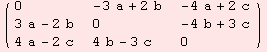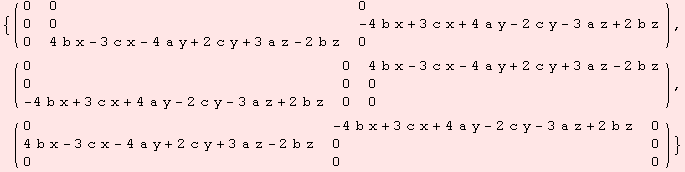The following line of code sets up the tri/multi-linear-form that represents the determinant of 3×3 matrices.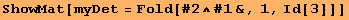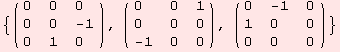Lets go two ways to obtain the determinant of the matrix X below.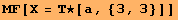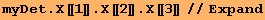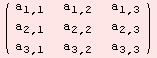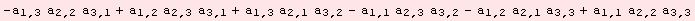Created by Mathematica  (September 30, 2006)